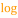# Logarithm

 Block Group: Math Operations Icon:The Logarithm block calculates the logarithm of a number using a specified base.

For information on using dataflow blocks, see Dataflow.

For accepted and excluded arguments of Math Operations blocks, see Math Operations.

## Input/Output Properties

The following properties of the Logarithm block can take input and give output.

• input (number)
• base (number)

input specifies the number to get the logarithm for.

base specifies the base for the logarithm.

## Output Property

The following property of the Logarithm block can give output but cannot take input.

• output (number)

output returns the logarithm for the specified input and base.

## Special Case for the Logarithm Block

A negative input value causes a NaN error result to be returned.

## Examples

The following image shows four examples of the Logarithm block.